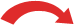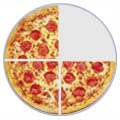# Using Rational Numbers

How to add, subtract, multiply and divide rational numbers

A rational number is a number that can be written as a simple fraction (i.e. as a ratio).

### Examples:

Number As a Fraction
5 5/1
1.75 7/4
.001 1/1000
0.111... 1/9

## In general ...

So a rational number looks like this:

pq

But q cannot be zero, as that is dividing by zero.

## How to Add, Subtract, Multiply and Divide

When the rational number is something simple like 3, or 0.001, then just use mental arithmetic, or a calculator!

But what about when it is in pq form?

 ½ Well, a rational number is a fraction, so we can use:

Here we will see those operations in a more general Algebra style.

You might also like to read Fractions in Algebra.

Let us start with multiplication, as that is the easiest.

## Multiplication

To multiply two rational numbers multiply the tops and bottoms separately, like this:

Here is an example:

## Division

To divide two rational numbers, first flip the second number over (make it a reciprocal) and then do a multiply like above:

Here is an example:

## Addition and Subtraction

We will cover Addition and Subtraction in one go, as they are the same method.

Before we add or subtract, the rational numbers should have the same bottom number (called a Common Denominator).

The easiest way to do this is to

Multiply both parts of each number by the bottom part of the other

Like this (note that the dot · means multiply):

Here is an example of addition:

And an example of subtraction (the middle step is skipped to make it quicker):

## Simplest Form

Sometimes we have a rational number like this:

1015

But that is not as simple as it can be!

We can divide both top and bottom by 5 to get:

 ÷ 51015   =   23÷ 5

Now it is in "simplest form", which is how most people want it!

## Be Careful With "Mixed Fractions"

We may be tempted to write an Improper Fraction (a fraction that is "top-heavy", i.e. where the top number is bigger then the bottom number) as a Mixed Fraction:

For example 7/4 = 1 3/4, shown here:Improper FractionMixed Fraction 7/4 1 3/4=But for mathematics the "Improper" form (such as 7/4) is actually better.

Because Mixed fractions (such as 1 3/4) can be confusing when we write them down in a formula, as it can look like a multiplication:

Mixed Fraction:   Improper Fraction: What is: 1 + 214 ? Is it: 1 + 2 + 14 = 314 ? Or is it: 1 + 2 × 14 = 112 ? What is: 1 + 94 ? It is: 44 + 94 = 134So try to use the Improper Fraction when doing mathematics.